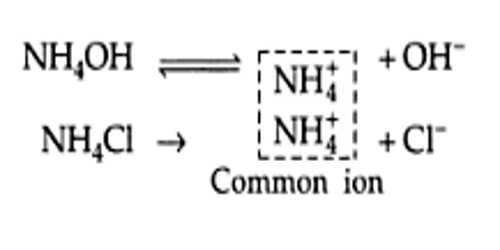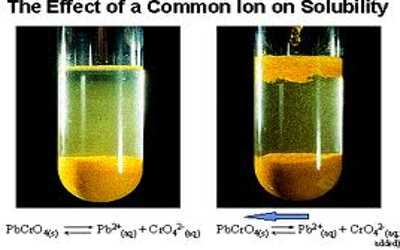Chemistry

# Solubility and Common Ion EffectSolubility and Common Ion Effect

We know that the dissociation of a weak acid is depressed when an electrolyte with an ion common to the ions formed by the acid is added to its solution. This happens because the added ion shifts the equilibrium to the side of the undissociated acid. A similar situation arises when an electrolyte with an ion common to a sparingly soluble salt is added to the saturated solution of the salt. The addition of the electrolyte decreases the solubility of the sparingly soluble salt. For example, if to a saturated solution of Ag2CrO4 some AgNO3 has added the solubility of Ag2CrO4 decreases. The effect, as in the case of weak acid, is known as the common ion effect. Let consider the equilibrium condition for a saturated solution of Pb(II) chromate:

PbCrO4 (s) + aq ↔ Pb2+ + CrO42- (aq)The Ksp for this ionic equilibrium has been found to be 1.8 x 10-14 at 25°C. At a particular temperature, the Ksp of this equilibrium depends only on the product of the concentrations of the constituent ions. i.e. [Pb2+] [CrO42-]. If, however, the concentration of either of the ions is increased at equilibrium, the concentration of the other must decrease correspondingly to maintain the constant value of Ksp. Suppose we add a soluble salt Na2CrO4 to the saturated solution of PbCrO4 (s). As a result, the concentration of the common ion CrO42- increases in the solution, and a portion of it will react with Pb2+ to form more solid PbCrO4 (s). The equilibrium will, therefore, shift to the left as shown below:

PbCrO4 (s) + (aq) ↔ Pb2+ (aq)+ CrO42- (aq)

In effect, the solubility of PbCrO4 (s) will be reduced. The same conclusions can be drawn if, instead of Na2CrO4, we add a soluble lead salt Pb(NO3)2. The added Pb2+ ions will combine with some CrO42- (aq) to decrease the amount of dissolved PbCrO4.